﻿ Miscellaneous > M-1 System Options > Sales Options 1 > Interest Calculation Methods

# Interest Calculation Methods

Navigation:  Miscellaneous > M-1 System Options > Sales Options 1 >

# Interest Calculation MethodsThis default option is accessed from the Sales Options 2 tab under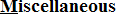>>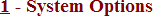There are three different interest calculation methods to select from in the system options. Some methods may not be available in specific states.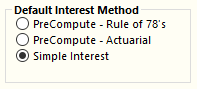PreCompute - The amount of Interest is Calculated at the Time of the Sale.   Rule of 78's - This is a quick & easy way to determine the amount of interest each payment includes. Let's assume there is a year-long loan with twelve monthly installments. Add together all the interest payments that are included in each payment (12 for the first installment, 11 for the second installment, etc). The total number is 78 for twelve installments (hence the name). The first installment pays 12/78 of the finance charge; the second installment pays 11/78 of the finance charge, etc. The mathematical representation of the number of interest payments is: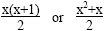Actuarial - Unlike the Rule of 78s, this method takes into account the number of days in the month when calculating the amount of interest in each payment. This is the pre-computed method most similar to simple interest.     Simple Interest - The amount of Interest Depends Upon When Payments are Made.   Interest is added daily based on the amount of principle balance of the account. The formula is: interest=principal x rate x time       The most noticeable difference between pre-computed and simple interest is what appears on the receipt printed after a payment is taken.     Because the amount of interest owed changes on a daily basis, the simple interest receipt will list separately the amount of the payment that was applied to the interest owed.  If the total payment was an amount more than the owed interest, the remainder of the payment will show as applied to the principal balance.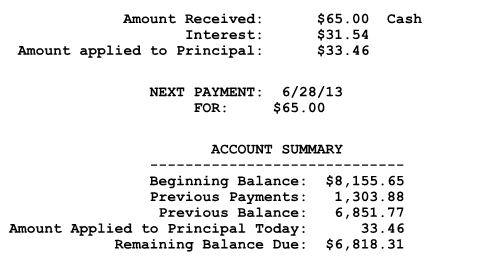Because the interest is already determined at the time of the sale, the pre-computed interest receipt will show the total contract balance after the entire amount of the received payment is subtracted.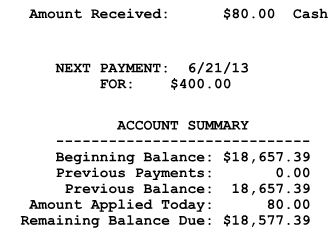Back to System Options

Used Car Dealer Software by Frazer Computing, Inc.